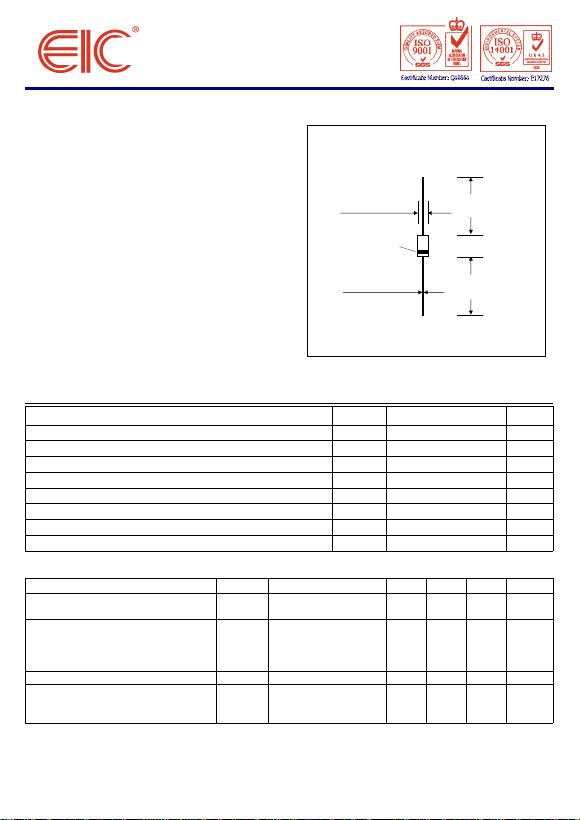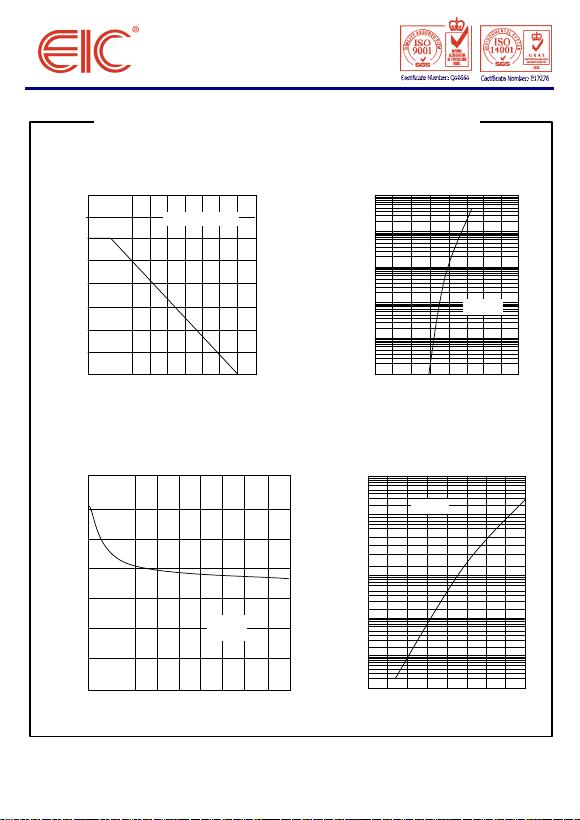1N914/A/B HIGH SPEED SWITCHING DIODES
FEATURES :
• High switching speed: max. 4 ns
• Continuous reverse voltage:max. 75 V
• Repetitive peak reverse voltage:max. 100 V
• Repetitive peak forward current: max. 225 mA
• Pb / RoHS Free
MECHANICAL DATA
:
Case: DO-35 Glass Case
Weight: approx. 0.13g
Maximum Ratin
g
s and Thermal Characteristics
(
Ratin
g
at 25 °C ambient temperature unless otherwise specified.
)
Symbol Value Unit
VRRM 100 V
VRM 75 V
Maximum Continuous Forward Current IF75 mA
Maximum Power Dissipation PD250 mW
Maximum Repetitive Peak Forward Current IFRM 225 mA
Maximum Non-repetitive Peak Forward Current at t = 1s IFSM 0.5 A
Maximum Junction Temperature TJ175 °C
Storage Temperature Range TS-65 to + 200 °C
Electrical Characteristics (TJ = 25°C unless otherwise noted)
Parameter Symbol Min Typ Max Unit
VR = 20 V - - 25 nA
VR = 20 V , Tj = 150 °C--50
μA
1N914 IF = 10 mA - - 1.0 V
1N914A IF = 20 mA - - 1.0 V
1N914B IF = 5 mA 0.62 - 0.72 V
1N914B IF = 100 mA - - 1.0 V
Diode Capacitance Cd - - 4.0 pF
IF = 10 mA to IR = 60 mA
RL = 100 Ω ; measured
at IR = 1mA
Page 1 of 2 Rev. 03 : March 25, 2005
Parameter
Maximum Repetitive Peak Reverse Voltage
Maximum Continuous Reverse Voltage
Reverse Current
ns4--
Test Condition
f = 1MHz ; VR = 0
Reverse Recovery Time Trr
IR
Forward Voltage VF
DO - 35 Glass
(DO-204AH)
0.079(2.0 )max.
0.150 (3.8)
max.
0.020 (0.52)max.
Dimensions in inches and
(
millimeters
)
1.00 (25.4)
min.
1.00 (25.4)
min.
Cathode
MarkRATING AND CHARACTERISTIC CURVES ( 1N914/A/B )
FIG. 1 MAXIMUM PERMISSIBLE CONTINUOUS FIG. 2 TYPICAL FORWARD VOLTAGE
FORWARD CURRENT AS A FUNCTION OF
AMBIENT TEMPERATURE.
FIG. 3 TYPICAL DIODE CAPACITANCE AS FIG. 4 TYPICAL REVERESE CURRENT
A FUNCTION OF REVERSE VOLTAGE VERSUS JUNCTION TEMPERATURE
Page 2 of 2 Rev. 03 : March 25, 2005
01020
Reverse Voltage , VR (V)
0.4
0.5
1.2
Diode Capacitance , Cd (pF)
0 0.4 1.2
Forward Voltage , VF (V)
0.01
10
1000
Forward Current , IF (mA)
0.1
1
100
00 100 200
Ambient Temperature , Ta (°C)
25
75
1.4
0.6
0.7
0.8
1.0
CONTINUOUS F ORWARD CURRENT,
IF (mA)
50
100
0.8
f = 1MHz;
TJ = 25°C